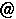## Turaev $B;a$NO"B39V5A(B

$B?tM}2r@O8&5f=j5R0w65 $B6=L#$N$"$kJ}$O!"$I$&$>$U$k$C$F$4;22C$/$@$5$$!#(B $BF|;~!'(B2006$BG/(B 2$B7n(B 22$BF|!J?e!K!A(B24$BF|!J6b!K!"3FF|$H$b(B 14$B;~!A(B17$B;~(B

$B>l=j!'5~ETBg3X(B $B?tM}2r@O8&5f=j(B 115$B9f<<(B $B!J(B$B@h$K%"%J%&%s%9$7$^$7$?9V5A<<$,JQ99$K$J$j$^$7$?(B$B!K(B

$B9V;U!'(BVladimir Turaev $B;a(B $B!J(BCNRS - Louis Pasteur University, Strasbourg / $B?tM}2r@O8&5f=j!K(B

Title: Topology of words

Abstract: Words are finite sequences of letters from a fixed alphabet. We study words using ideas and techniques from low-dimensional topology. The relevance of topology is suggested by the well-known connection to closed plane curves, first pointed out by Gauss, and also by the phenomenon of linking of letters in words. A prototypical example is provided by the words $abab$ and $aabb$. The letters $a,b$ are obviously linked in the first word and unlinked in the second one. This phenomenon is similar to the linking of geometric objects, for instance knots in Euclidean 3-space. The study of words by topological methods includes as special cases a study of closed cuves and link diagrams on oriented surfaces. A number of standard link invariants, say the link quandle, the Kauffman bracket polynomial, the genus, naturally appear in this setting although in a modified form.

Contents.
1. Words and etale words.
2. Nanowords.
3. Homotopy of nanowords.
4. Curves and links on surfaces as nanowords.
5. Self-linking and coverings of nanowords.
7. Analysis litterae: homotopy classification of words of length $\leq 5$.
$BO"Mm@h!'BgDP(B $BCNCi(B $B!J5~Bg?tM}8&!"(Btomotadakurims.kyoto-u.ac.jp$B!K(B